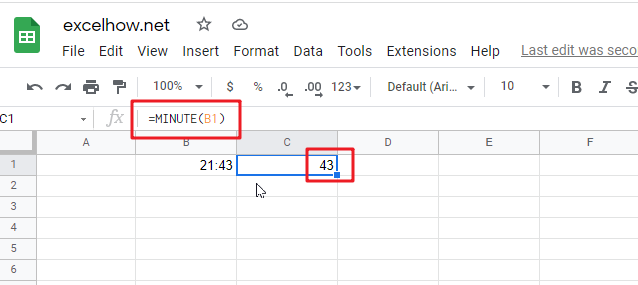# ExcelHow

This post will guide you how to use Google Sheets MINUTE function with syntax and examples.

## Description

The Google Sheets MINUTE returns the minutes of a time value. And the minutes is an integer number from 0 to 59. So you can use the MINUTE function to extract the minute component of a given time value.

The MINUTE function can be used to extract the minute component of a given time as a number between 0-59 in google sheets. The purpose of this function is to get minute as a number from a time and its returned values is a number between 0 and 59.

For example, for a valid time value 10:29 AM, the MINUTE function will return 29. So you can pass the returned result into another formula.

The MINUTE function is a build-in function in Google Sheets and it is categorized as a Date function.

## Syntax

The syntax of the MINUTE function is as below:

=MINUTE (time)

Where the MINUTE function arguments is:

• time -This is a required argument. A valid time value that contains the minute you want to extract. The times can be entered as a text strings within quotation marks (for example, “10:32 AM“), or typed as a decimal numbers, or the results of others formulas. It must be a cell reference that containing a date or time, or a function that returning a date/time type or a number.

Note:

• Time values are a portion of a date value and represented by a decimal number (for example, 12:00 PM is represented as 0.5, since it is half of a day).
• If the `time` is not a valid Excel time, it will return #VALUE! Error.
• `Time` argument can be supplied to the MINUTE function as a time text string or as a decimal numbers. If you wish to create a time value with separate hour, minute and second, you can use the TIME function.

## Google Sheets MINUTE Function Examples

The below examples will show you how to use google sheets MINUTE Function to return an integer number that representing the minute component of a given time.

#1 get minute portion of the time, enter the following formula in Cell C1.

`=MINUTE(B1)`